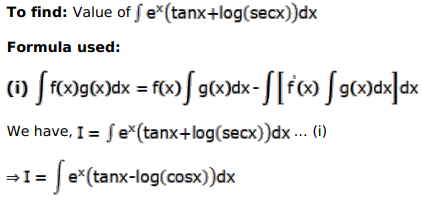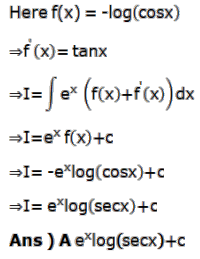# Mark against the correct answer in each of the following:

Question:

Mark $(\sqrt{)}$ against the correct answer in each of the following:

$\int e^{x}(\tan x+\log \sec x) d x=?$

A. $e^{x} \log \sec x+C$

B. $e^{x} \tan x+c$

C. $e^{x}(\log \cos x)+C$

D. none of these

Solution: